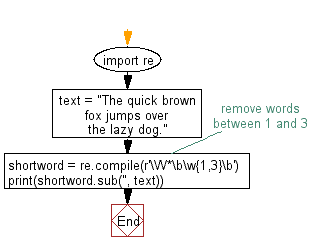﻿ Python: Remove words from a string of length between 1 and a given number - w3resource# Python: Remove words from a string of length between 1 and a given number

## Python Regular Expression: Exercise-49 with Solution

Write a Python program to remove words from a string of length between 1 and a given number.

Sample Solution:-

Python Code:

``````import re
text = "The quick brown fox jumps over the lazy dog."
# remove words between 1 and 3
shortword = re.compile(r'\W*\b\w{1,3}\b')
print(shortword.sub('', text))
```
```

Sample Output:

```quick brown jumps over lazy.
```

Pictorial Presentation:Flowchart:## Visualize Python code execution:

The following tool visualize what the computer is doing step-by-step as it executes the said program:

Python Code Editor:

Have another way to solve this solution? Contribute your code (and comments) through Disqus.

What is the difficulty level of this exercise?

Test your Python skills with w3resource's quiz

﻿

## Python: Tips of the Day

Creating a sequence of numbers (zero to ten with skips):

```>>> range(0,10,2)
[0, 2, 4, 6, 8]
```